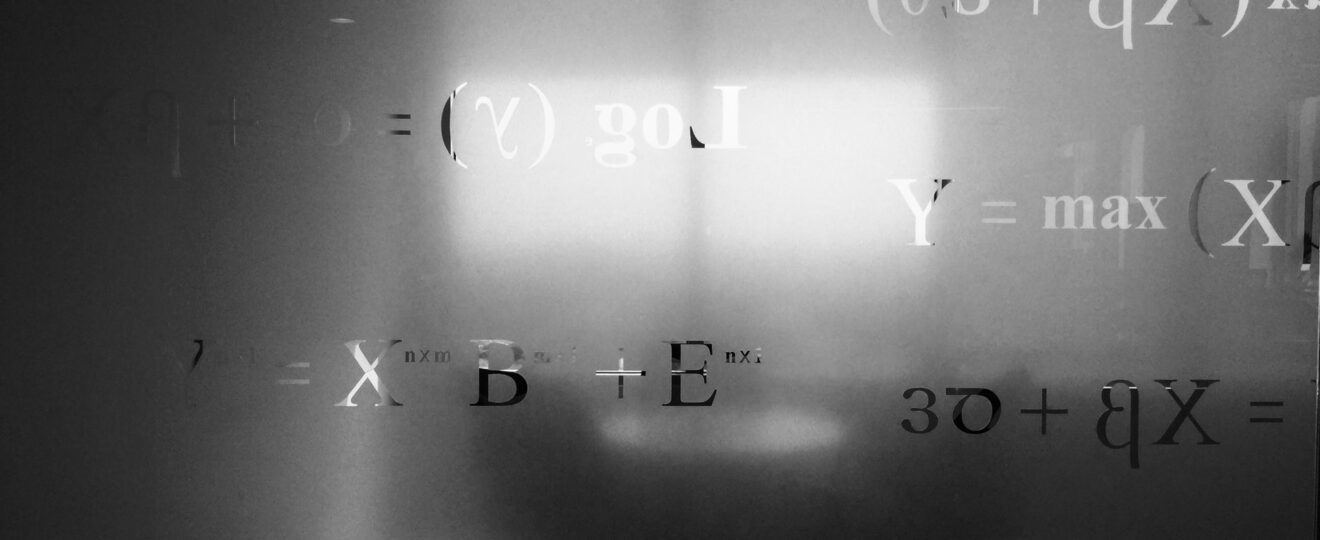Education

# Basic Concepts About Percentage Formula And Percentage ChangeIn mathematics, the percentage is defined as the number or ratio that is expressed as a fraction of 100. To calculate the percentage of a number, the value is divided by whole and then multiplied by 100. Here through this article, one can learn about the percentage formula and percentage change.

## Formula to Calculate the Percentage of a Number

The percentage formula in mathematics is used to calculate the share of something in terms of 100. The percentage is referred to as a ratio or fraction where the value of the whole is always 100. The symbol which is used to denote percentage is %, also called the percent symbol. It can be computed by dividing the value by the total value and then multiplying the computed value by 100.

Percentage = Value/Total value × 100

Here, ‘value’ refers to any given value being part of the total value.

## How to Compute the Percentage of a Number?

To calculate the percentage, let us consider the value to be 60 and the total value to be 120.

The below-given procedure is to be followed to calculate the percentage of a number:

1. Divide the value by the given total value.

Value/Total value = 60/120 = ½

2.      Now multiply the computed value by 100.

Value/Total value × 100 = 1/2 × 100 = 50%

Therefore, the percentage of a given number is 50%

## Applications of the Percentage Formula

Following are some real-life applications of the percentage formula:

• The value of prices, grades, etc is compared.
• Simple interest, compound interest, depreciation, rate of growth is calculated with the help of a percentage formula.
• Problems related to profit and loss, discount, etc., are solved.
• Percentage of nutrients given on the packaging of food items.
• Composition of different gases such as oxygen, nitrogen, carbon dioxide, and many more in the air.

## Meaning of Percentage Change

Percentage change is also known as the percentage change of a quantity. It refers to the ratio of the difference in the quantity to its initial value multiplied by 100. The change in the percentage takes place when the percent of its initial quantity is either increased or decreased to obtain its final value. Therefore, there are two types of percentage change:

• Percentage Increase
• Percentage decrease

## Percentage Change Formula

Percentage change is calculated by subtracting the old value from the new value and then dividing by the old value and in the last final value computed is multiplied by 100. The percentage formula in the terms of mathematics is as follows:

Percentage change = Final Value – Initial Value/ initial Value × 100

## Calculation of Percentage Change

Let us consider, initial value to be 40 and the final value to be 80.

The below-given procedure is to be followed when calculating the percentage change:

1. In the first step, the initial value is subtracted from the final value.

Final Value – Initial Value = 80 – 40 = 40

2.      Now that the absolute value is computed, divide it by the given initial value.

Final Value – Initial Value/Initial Value = 40/40 = 1

3.  In the final step, the result is multiplied by 100.

Final Value – Initial Value/Initial Value × 100 = 1 × 100 = 100%

Therefore, the percentage change (percentage increase) is 100%

## Bottom Line

Cuemath is an educational company offering math online classes so that students at their convenience can understand the complicated concepts. This platform is working hard to provide world-class knowledge to the students by hiring teachers who can make math more of a fun and interesting subject.